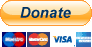# B4X: ABMaterials B4JS – 01 Getting Started

In this first tutorial, we are going to create a killer method to calculate the distance between two GEO locations. There are better ways to do so, but for demos sake…

Creating a B4JS class is very simple. Just use the menu in B4J and add a Standard Class.

```'Class module
Sub Class_Globals

End Sub

Public Sub Initialize

End Sub
```

First thing we MUST do is renaming the Initialize method to InitializeB4JS. This is because the transpiler uses this method name to determine it has to translate this class to JavaScript.

This InitializeB4JS method can NOT have parameters!

Now we can start adding our code, just as usual in B4J. As almost the whole Core library of B4J is available, this is pretty forward. Except from the ABM methods, this could easily be seen as B4J.

```'Class module
Sub Class_Globals
' use public or dim if you want to share this variable over ALL B4JS classes
' use private if only within this class
Dim ToKM As Double = 1.609344
Dim ToMiles As Double = 0.8684

' to access the constants
Public ABM As ABMaterial 'ignore
' so we can use an msgbox
Public Page As ABMPage 'ignore, just to be able to run ABMPage functions
End Sub

'Initializes the object. You can NOT add parameters to this method.
'MUST be called InitializeB4JS is automatically called when using this class
Public Sub InitializeB4JS

End Sub

public Sub CalcDistance(Lat1 As Double, Lon1 As Double, Lat2 As Double, Lon2 As Double, Unit As String)
Dim theta As Double
Dim Distance As Double
theta = Lon1 - Lon2
' logging some intermediate value
Log("Distance = " & Distance)
Distance = ACos(Distance)
' logging some intermediate value
Log("Distance = " & Distance)
Distance = Distance * 60 * 1.1515
' if we would use Page.Msgbox here, we would see in the logs an error: msgbox is NOT supported in B4JS!
' we must use the B4JS equivalent method Page.B4JSMsgbox
Select Case Unit.ToUpperCase
Case "K"
Page.B4JSMsgbox("msgbox", "The distance is " & (Distance * ToKM) & " kilometers!", "Tutorial", "OK", False, ABM.MSGBOX_POS_CENTER_CENTER, "")
Case "N"
Page.B4JSMsgbox("msgbox", "The distance is " & (Distance * ToMiles) & " miles!", "Tutorial", "OK", False, ABM.MSGBOX_POS_CENTER_CENTER, "")
Case Else
Page.B4JSMsgbox("msgbox", "No idea what you are doing :-)", "Tutorial", "OK", False, ABM.MSGBOX_POS_CENTER_CENTER, "")
End Select
End Sub

' some helper methods
Sub deg2rad(Deg As Double) As Double
Return Deg * cPI / 180
End Sub

Return Rad * 180 / cPI
End Sub
```

VERY IMPORTANT!!!
Depending on how you declare the variable in Class_Globals, a variable is shared between class instances or not:

This concept becomes very important when we start using ABMComponents because when you attach a B4JSOn… event to an ABMComponent, it gets its own instance of your class. The Public/Dim variables will be shared among all the components using this B4JS Class

To use our new method, I’ll make a button in ConnectPage() on the ABM page (this will be explained in a future tutorial) and when we click, we do a calculation:

```Dim btn As ABMButton
btn.InitializeFlat(page, "btn", "", "", "Calculate", "")
' B4JSUniqueKey is explained in a later turorial
btn.B4JSUniqueKey = "btn001"
' define the B4JS OnClickedEvent
btn.B4JSOnClick("B4JSCalculateDistance", "CalcDistance", Array As Object(32.9697, -96.80322, 29.46786, -98.53506, "K"))
```

Alternative, not using an ABMButton but calling our method directly:

```page.B4JSRunMethod("B4JSCalculateDistance", "CalcDistance", Array As Object(32.9697, -96.80322, 29.46786, -98.53506, "K"))
```

So let’s check our results (click to enlarge):1. In the browsers log we see our two intermediate log() calls.
2. the solution to our call is shown in a message box.

But in the B4J log we also see that the normal btn_Click event is raised! That is not what we want.

To avoid this, we make a simple change to our CalcDistance method. We return a boolean true: this is saying ‘consume the click on the browser side and don’t go to the server‘.

```public Sub CalcDistance(Lat1 As Double, Lon1 As Double, Lat2 As Double, Lon2 As Double, Unit As String) As Boolean
...
' important, if we do not want to raise the servers btn_click events, we must return true
Return True
End Sub
```

And hooray, our server is not contacted any more!Ultimate proof we are not contacting the server for this code. I’ve stopped the server app and I can still use the button:This concludes the first tutorial.

For those interested in the JavaScript generated for our class, here it is:

```var_tokm=1.609344;
var _tomiles=0.8684;
var _abm;
var _page;
function b4js_b4jscalculatedistance() {
var self;
this.initializeb4js=function(){
self=this;
try {
}
catch(err) {
console.log(err.message + ' ' + err.stack);
}
};
this.calcdistance=function(_lat1,_lon1,_lat2,_lon2,_unit){
try {
var _theta=0;
var _distance=0;
_theta = _lon1-_lon2;
console.log("Distance = "+_distance);
_distance = (Math.acos(_distance));
console.log("Distance = "+_distance);
_distance = _distance*60*1.1515;
switch ("" + _unit.toUpperCase()) {
case "" + "K":
var _b4js_returnname="msgbox";
b4js_msgbox("default","Tutorial","The distance is "+(_distance*_tokm)+" kilometers!","OK",'swal2pos-cc', false);;
break;
case "" + "N":
var _b4js_returnname="msgbox";
b4js_msgbox("default","Tutorial","The distance is "+(_distance*_tomiles)+" miles!","OK",'swal2pos-cc', false);;
break;
default:
var _b4js_returnname="msgbox";
b4js_msgbox("default","Tutorial","No idea what you are doing :-)","OK",'swal2pos-cc', false);;
break;
}
self.testjson();
callAjax("https://jsonplaceholder.typicode.com/posts/1","GET","jsonp", "","myJob1", true,"b4jscalculatedistance");
return true;
}
catch(err) {
console.log(err.message + ' ' + err.stack);
}
};

try {
return _deg*Math.PI/180;
}
catch(err) {
console.log(err.message + ' ' + err.stack);
}
};
try {
}
catch(err) {
console.log(err.message + ' ' + err.stack);
}
};
}
```

In the text tutorial we are going to see how we can use inline JavaScript snippets within our B4JS classes!

Alwaysbusy

Click here toand support ABMaterial